Boost C++ Libraries

...one of the most highly regarded and expertly designed C++ library projects in the world.

Class template uniform_smallint

boost::random::uniform_smallint

Synopsis

template<typename IntType = int>
class uniform_smallint {
public:
// types
typedef IntType input_type;
typedef IntType result_type;

// member classes/structs/unions

class param_type {
public:
// types
typedef uniform_smallint distribution_type;

param_type(IntType = 0, IntType = 9);

IntType a() const;
IntType b() const;

template<typename CharT, typename Traits>
friend std::basic_ostream< CharT, Traits > &
operator<<(std::basic_ostream< CharT, Traits > &, const param_type &);
template<typename CharT, typename Traits>
friend std::basic_istream< CharT, Traits > &
operator>>(std::basic_istream< CharT, Traits > &, const param_type &);
friend bool operator==(const param_type &, const param_type &);
friend bool operator!=(const param_type &, const param_type &);
};

explicit uniform_smallint(IntType = 0, IntType = 9);
explicit uniform_smallint(const param_type &);

result_type a() const;
result_type b() const;
result_type min() const;
result_type max() const;
param_type param() const;
void param(const param_type &);
void reset();
template<typename Engine> result_type operator()(Engine &) const;
template<typename Engine>
result_type operator()(Engine &, const param_type &) const;

template<typename CharT, typename Traits>
friend std::basic_ostream< CharT, Traits > &
operator<<(std::basic_ostream< CharT, Traits > &,
const uniform_smallint &);
template<typename CharT, typename Traits>
friend std::basic_istream< CharT, Traits > &
operator>>(std::basic_istream< CharT, Traits > &,
const uniform_smallint &);
friend bool operator==(const uniform_smallint &, const uniform_smallint &);
friend bool operator!=(const uniform_smallint &, const uniform_smallint &);
};

Description

The distribution function uniform_smallint models a random distribution . On each invocation, it returns a random integer value uniformly distributed in the set of integer numbers {min, min+1, min+2, ..., max}. It assumes that the desired range (max-min+1) is small compared to the range of the underlying source of random numbers and thus makes no attempt to limit quantization errors.

Letthe desired range of integer numbers, and letbe the range of the underlying source of random numbers. Then, for the uniform distribution, the theoretical probability for any number i in the rangewill be. Likewise, assume a uniform distribution onfor the underlying source of random numbers, i.e.. Letdenote the random distribution generated by uniform_smallint. Then the sum over all i inofshall not exceed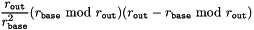.

The template parameter IntType shall denote an integer-like value type.

NoteThe property above is the square sum of the relative differences in probabilities between the desired uniform distributionand the generated distribution. The property can be fulfilled with the calculation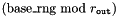, as follows: Let. The base distribution onis folded onto the range. The numbers i < r have assigned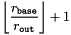numbers of the base distribution, the rest has only. Therefore,for i < r andotherwise. Substituting this in the above sum formula leads to the desired result.

Note: The upper bound foris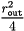. Regarding the upper bound for the square sum of the relative quantization error of, it seems wise to either chooseso that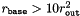or ensure thatis divisible by.

uniform_smallint public construct/copy/destruct

1. explicit uniform_smallint(IntType min = 0, IntType max = 9);

Constructs a uniform_smallint. min and max are the lower and upper bounds of the output range, respectively.

2. explicit uniform_smallint(const param_type & param);

Constructs a uniform_smallint from its parameters.

uniform_smallint public member functions

1. result_type a() const;

Returns the minimum value of the distribution.

2. result_type b() const;

Returns the maximum value of the distribution.

3. result_type min() const;

Returns the minimum value of the distribution.

4. result_type max() const;

Returns the maximum value of the distribution.

5. param_type param() const;

Returns the parameters of the distribution.

6. void param(const param_type & param);

Sets the parameters of the distribution.

7. void reset();

Effects: Subsequent uses of the distribution do not depend on values produced by any engine prior to invoking reset.

8. template<typename Engine> result_type operator()(Engine & eng) const;

Returns a value uniformly distributed in the range [min(), max()].

9. template<typename Engine>
result_type operator()(Engine & eng, const param_type & param) const;

Returns a value uniformly distributed in the range [param.a(), param.b()].

uniform_smallint friend functions

1. template<typename CharT, typename Traits>
friend std::basic_ostream< CharT, Traits > &
operator<<(std::basic_ostream< CharT, Traits > & os,
const uniform_smallint & ud);

Writes the distribution to a std::ostream.

2. template<typename CharT, typename Traits>
friend std::basic_istream< CharT, Traits > &
operator>>(std::basic_istream< CharT, Traits > & is,
const uniform_smallint & ud);

Reads the distribution from a std::istream.

3. friend bool operator==(const uniform_smallint & lhs,
const uniform_smallint & rhs);

Returns true if the two distributions will produce identical sequences of values given equal generators.

4. friend bool operator!=(const uniform_smallint & lhs,
const uniform_smallint & rhs);

Returns true if the two distributions may produce different sequences of values given equal generators.